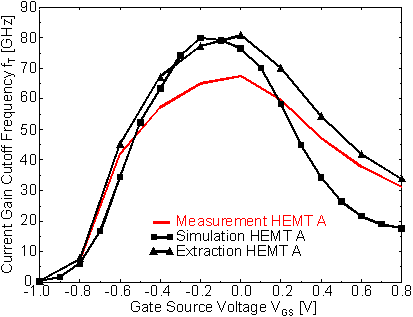Next: 6.1.2.1 Reduction of the Gate Length Up: 6.1 Low Noise HEMT Previous: 6.1.1.2 Comparison of HEMT A and HEMT C

6.1.2 RF Characteristics

One of the most important figure of merit of a HEMT is the current-gain cutoff frequency fT given by (10) and fmax given by (11) as described in Chapter 3.

For the case of HEMT AFigure 6.13 shows a comparison of the functions fT (VGS) determined in three different ways: i) measured (i. e., obtained by S­parameter measurements), ii) calculated with (10) where gmi, CGSi, and CGDi were obtained from extractions using the small-signal equivalent network shown in Figure 3.1, and iii) obtained from simulations also using (10) with the approximation for the extrinsic values described in Chapter 3.Figure 6.13 Measured (bold line without symbols) and calculated fT with simulated (squares) and extracted (triangles) CG and gm at VDS = 2.0 V

The last two cases offer the possibility to determine the device performance excluding the contacting network which is necessary for circuit design.

The maximum fT of the simulation was fitted to fT max calculated with extracted small signal parameters by adjusting the cross section of the T­gate. The smaller simulated fT's below and above gm max reflect the underestimated electron velocity due to the DD model in the GaAs and AlGaAs layers. The measured maximum fT is about 13 GHz lower than the calculated ones mainly due to the presence of the parasitic elements of the small signal equivalent shown in Figure 3.1. The values of the small-signal circuit elements for HEMT A obtained by parameter extraction, which are considered to be not bias dependent, are given in  Table 6.4.

 CPG+CPD [fF] LG [pH] LD [pH] LS [pH] RG [W] RD [W] RS [W] 25 16.5 13.1 3.0 1.4 3.4 2.3

In the following we want to examine the influence of the geometrical parameters LG and LR on the magnitude of fT and fmax simulated according to (10) and (11), respectively. The capacitances CGS and CGD entering the equations are considerably influenced not only by LG and LR but also by the shape of the gate metal cross section (imagine, for instance, the case of a T-gate) and the dielectric constant er of the passivation material that fills the space between metal structure and semiconductor surface. The simulator is able to take all these effects of surface topology fully into account. On the other hand, only in simulation it is possible to analyze the hypothetical case that the gate does not interact capacitively with the surrounding semiconductor surfaces or with the neighboring ohmic contacts. This can be achieved by choosing er = 0 and leads to the determination of the theoretical maximum of fT for given LG and LR.Next: 6.1.2.1 Reduction of the Gate Length Up: 6.1 Low Noise HEMT Previous: 6.1.1.2 Comparison of HEMT A and HEMT C

Helmut Brech
1998-03-11